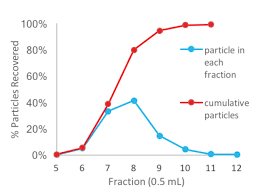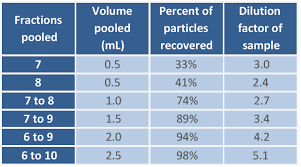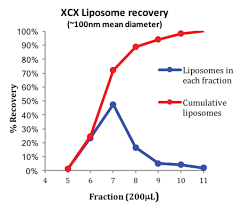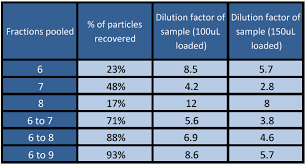Izon Science Support Centre

# Are samples diluted when passed through the qEV columns?

Yes. We have carried out studies with known concentrations of both polystyrene nanoparticle standards, and liposomes designed to mimic the size and composition of EVs.

qEVoriginal columns

The dilution factor depends on which fractions are pooled. Using a 500 μL starting volume of polystyrene standard particles (mode diameter 400 nm) the total particle recovery was ~100%. Figure 1 illustrates that fractions 5 to 11 need to be pooled to recover 100% of the particles. This results in a dilution factor of 7 and the collected fractions will have higher levels of contaminating protein so is not practical.Figure 1: Elution profile for 400 nm polystyrene particles.

Table 1 shows the dilution factor, and the recovery rate for various fraction collections. Whilst it is possible to recover 100% of particles, the highest concentration can be obtained by measuring each fraction and using the two fractions with highest EV and lowest protein concentration. Thus there is a compromise between recovery and purity of the EVs collected. Liposome trials have shown recovery rates of ~50%, when the two fractions 7 and 8 are pooled, which is consistent with the polystyrene example above.Table 1: Dilution effects as a parameter of fractions pooled and recovery rate.

Depending on the final concentration of EVs, fractions can be measured on a qNano with no dilution. For qNano measurements, 500-1600 particles/minute is an optimum rate. Samples that have rates exceeding this should be diluted. When the qEVoriginal columns are used according to this protocol and the fraction volumes are accurate, the EVs elute consistently in fractions 7, 8 and 9 with low levels in fractions 10 and 11.

qEVsingle columns

Using a 100 μL starting volume of liposome mimics (mean diameter 100 nm) the total particle recovery was ~100%. Figure 2 illustrates that fractions 6 to 11 need to be pooled to recover close to 100% of the particles. The dilution factor depends on which fractions are pooled. Pooling fractions 6 to 11 results in a dilution factor of 12.1 and the pooled fractions will have higher levels of contaminating protein which is not desirable.Figure 2: Elution profile of liposome mimics.

Table 2 shows the recovery rate and dilution factor for various fractions collected. Whilst it is possible to recover 100% of particles, the highest concentration can be obtained by measuring each fraction and using the first one or two fractions with the highest EV and lowest protein concentration. Thus there is a compromise between recovery and purity of the EVs collected. The liposome mimic experiment shows a recovery rate of ~71%, when the two fractions 6 and 7 are pooled, with a dilution factor of 5.6. If 150 μL of sample is loaded the dilution can be reduced to 3.8 with minimal protein contamination.

When the qEV columns are used according to this protocol and the fraction volumes are accurate, the EVs elute consistently in fractions 6, 7 and 8 with low levels in fractions 9 and 10.Table 2: Dilution effect as a parameter of fractions pooled and recovery rate.